# Portfolio optimization with numerical optimizers and constraints

I’ll take the example of FAANG companies to find an ideal portfolio allocation over the past five years with different methods.

Modern Portfolio Theory is a hypothesis put forth by Harry Markowitz was based on the idea that a risk-averse investor can construct a portfolio to maximize expected return based on a given level of market risk. This results in the “efficient frontier formulation”.

I’ll start with a simple test case where I have five companies,FAANG, and I calculate the risk(expected variance in the portfolio) and return (expected annualised daily log returns) for time-series data from 06–05–2015 to 0–6–01–2020. I choose the “Adjusted Close” values provided by Yahoo Finance but these can be changed to any value one might prefer.

So, the question is, what are the ideal weights for each company over this time-period. For simplicity, I’ll keep the weights constant over time for now.

# Data Gathering: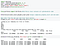Example of how I handle the data. I use only the Adj Close values, but any other value can, in principle, be extracted.

The Portfolio class uses pandas datareader and Quandl/Yahoo APIs to download stock data, and populate one multi-index Pandas dataframe.

# Random allocation:

The simplest way to find reasonably optimal weights is to randomly allocate them randomly, calculate risk, return and the ratio, and keep the best answer. For a portfolio comprising only five stocks, I think this is a simple but pretty decent strategy. Below is the a plot for 10⁶ points plotted with a random weight allocation between 0 and 1 for each stock.

The green dot is the portfolio with the highest Sharpe Ratio found after a million iterations.

Best Sharpe Ratio: 1.1848
Weights for best Sharpe ratio: 0.014, 0.009, 0.924, 0.041 and 0.011 for F, Apple, Amazon, N, G respectively. This says that putting 92.4% of the money in Amazon and 4% of the money in Netflix over the last 5 years would have been the best risk/return strategy.

With the future in mind, one would like to put constraints on how much to own any given stock. We also see a clear formation of the “efficient frontier” while using random values.

# Numerical optimization:

A faster way to arrive at the same result is to perform numerical optimization in stead of the guessing game in the earlier strategy. There are many numerical optimizers implemented in the Python SciPy library, originally from the fantastic Numerical Recipes books. In biophysics, we often combine the Monte-Carlo guesswork described above with more involved optimizers which require Jacobian and Hessian information such as Gradient Descent and L-BFGS (called Monte-Carlo Simulated Annealing).

I maximize the Sharpe ratio (minimize the negative) with the only constraint that all weights of add up to 1 and the bound on each weiht isbetween 0 and 1. Bounds can be changed for each stock individually, as I discuss below.

The ideal allocation turns out as:

Optimal weights: [0.0 0.018 0.915 0.067 0.0],
Best Sharpe ratio:1.1871

So, both methods give similar Sharpe ratios of 1.184 and 1.187 with a major allocation of 91–92% to Amazon. Exposing a portfolio to so much of one stock comes with its own risks. Thus, I constrain my allocation to the Amazon stock and see how this effects the returns, volatility and the Sharpe ratio.

Adding constraints: I generated optimal weights with a Sequential Least Squares programming algorithm which uses the Han–Powell quasi–Newton method with a BFGS update of the B–matrix and an L1–test function in the step–length algorithm.

I constraint the maximium weight given to the AMZN stock in the portfolio. Ten optimal portfolios constructed with this optimization are shown below.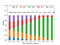Each stacked bar graph represents an optimal portfolio for the max. weight of Amazon. The Sharpe ratio for each portfolio is shown on top of the graph. A max Sharpe ratio was 1.187.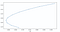The efficient frontier plotted for the optimal portfolio. Weight on amazon stock is constrained.

With a zero weightage for Amazon, a more equitable distribution can be seen in the optimal portfolio (33% Netflix, 26% Google, 28% Apple and 13% Facebook, but the Sharpe ratio has gone down from 1.187 to 0.884.).

Now, let’s try the same code by adding 5 more stocks to our original FAANG portfolio to see if the Sharpe Ratio differs.

Here’s a basket of 10 popular tech companies that I randomly picked out.
[“FB”,”AAPL”,”AMZN”,”NFLX”,”GOOG”,”NVDA”,”AMD”,”MSFT”,”MU”,”IBM”]

The Sharpe ratio has gone up to 1.421 at annualised returns of 43.7% with volatility of 0.3 due to significant contributions from AMD and Microsoft in particular.

Optimal weights are as follows:
0.000, 0.000, 0.474, 0.000,0.000, 0.270, 0.129, 0.126,0.000 and 0.000

showing that the major contributor still remains Amazon at 47.3%, followed by NVIDIA at 27%, AMD at 12.98% and Microsoft at 12.58% and every other company at basically 0! A similar analysis as above constraining the contribution of Amazon is shown below.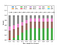Each stacked bar graph represents an optimal portfolio for the max. weight of Amazon. The Sharpe ratio for each portfolio is shown on top of the graph. A maximum Sharpe ratio of 1.421 is found. Of the 10 stocks in the list, adding Microsoft and AMD to the F,A,A,N,G portfolio led to a rise in the Sharpe ratio to 1.421 from 1.187.

To take this a bit further, and ensure that the code is generalisable, I take the top 50 stocks by Market Cap (and AMD) and re-run to see how high a Sharpe Ratio can one really get. With all 50 companies in the basket, the max. Sharpe Ratio has increased from 1.421 to 1.434. That really is not much of an increase!

The optimal allocation becomes: Amazon:40.2%, Walmart:7.9%, NVDIA: 22.4%, ADOBE:0.5%, MCDs:9.1%, Eli Lilly: 5.9%, Thermo Fisher: 3.4% and AMD and 10.5%. Only 8 stocks out of the 51 included in the basket contribute to the best Sharpe Ratio for an annualized return of 36.9% and a volatility of 0.262.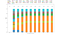Portfolios obtained after optimising a basket of 50 stocks. If we limit the ownership of each company to a maximum of 10%, then a Sharpe ratio of 1,.355 is the maximum possible. Without constraints, the max. Sharpe ratio found is 1.434.

Side notes:

1. What would be really nice is to have an exponential distribution of numbers that add up to the same constant value in order to generate as many distinct starting points as possible. Are these uniformly distributed? I don’t know!

Disclaimer: I’ve recently developed an interest in computational finance (portfolio optimisation methods) and this is really an excercise to test some python code I’ve been writing.

Optimising complex systems

## More from Sridhar N

Optimising complex systems